Question

# 9 of 28 A Review Constants | Periodic Table 2HCl(aq) + Na2CO3(aq) +2NaCl(aq) + H2O(l) +...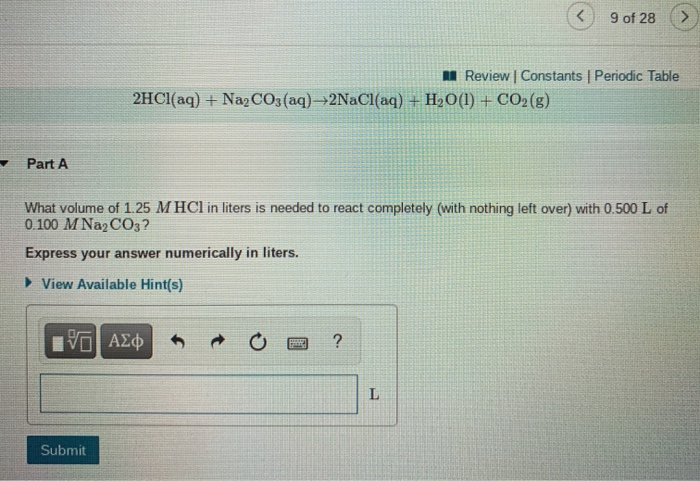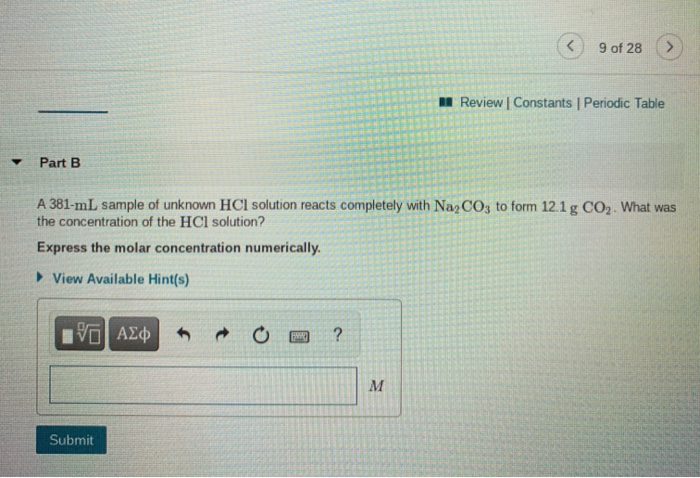9 of 28 A Review Constants | Periodic Table 2HCl(aq) + Na2CO3(aq) +2NaCl(aq) + H2O(l) + CO2(g) Part A What volume of 1.25 M HCl in liters is needed to react completely (with nothing left over) with 0.500 L of 0.100 M Na2CO3? Express your answer numerically in liters. ► View Available Hint(s) ΟΙ ΑΣΦ ? L Submit
9 of 28 Review | Constants Periodic Table Part B A 381-mL sample of unknown HCl solution reacts completely with Na2CO3 to form 12.1 g CO2. What was the concentration of the HCl solution? Express the molar concentration numerically. View Available Hint(s) IVO AXO ? M Submit

We need at least 10 more requests to produce the answer.

0 / 10 have requested this problem solution

The more requests, the faster the answer.

All students who have requested the answer will be notified once they are available.

#### Earn Coins

Coins can be redeemed for fabulous gifts.

Similar Homework Help Questions
• ### 2559b3823f31 > 8 of 28 A Review Constants Periodic Table ✓ Correct Part B What is...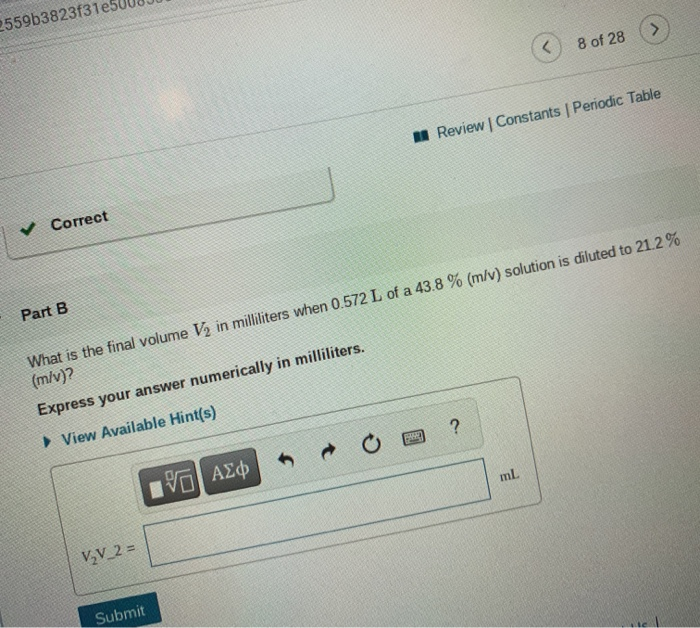2559b3823f31 > 8 of 28 A Review Constants Periodic Table ✓ Correct Part B What is the final volume V2 in milliliters when 0.572 L of a 43.8 % (m/v) solution is diluted to 21.2% (m/v)? Express your answer numerically in milliliters. View Available Hint(s) ? ml IVO AXO V₂V_2= Submit 8 of 28 M Review | Constants Periodic Table Part C A 554 ml NaCl solution is diluted to a volume of 1.08 L and a concentration of 2.00...

• ### Solution Stoichiometry Hydrochloric acid (HCl) reacts with sodium carbonate (Na2CO3), forming sodium chloride (NaCl), water (H2O),...

Solution Stoichiometry Hydrochloric acid (HCl) reacts with sodium carbonate (Na2CO3), forming sodium chloride (NaCl), water (H2O), and carbon dioxide (CO2). This equation is balanced as written: 2HCl(aq)+Na2CO3(aq)→2NaCl(aq)+H2O(l)+CO2(g) a) What volume of 2.75 M HCl in liters is needed to react completely (with nothing left over) with 0.750 L of 0.300 M Na2CO3? b) A 565-mL sample of unknown HCl solution reacts completely with Na2CO3 to form 10.1 g CO2. What was the concentration of the HCl solution? How do I...

• ### Part A What volume of 1.00 M HCl in liters is needed to react completely (with...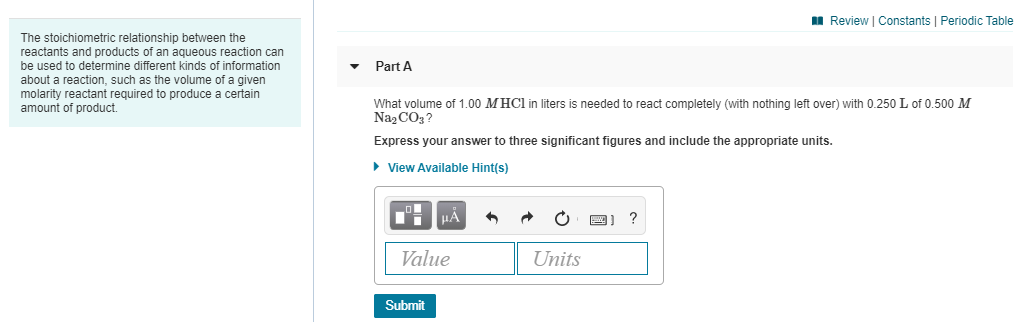Part A What volume of 1.00 M HCl in liters is needed to react completely (with nothing left over) with 0.250 L of 0.500 M Na2CO3? Express your answer to three significant figures and include the appropriate units. Part B A 419-mL sample of unknown HCl solution reacts completely with Na2CO3 to form 12.1 g CO2. What was the concentration of the HCl solution? Express your answer to three significant figures and include the appropriate units. A Review Constants Periodic...

• ### 3 of 28 > A Review Constants Periodic Table Part A You have prepared a saturated...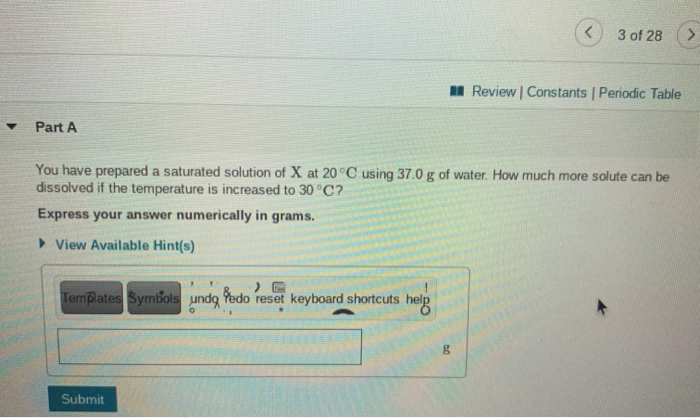3 of 28 > A Review Constants Periodic Table Part A You have prepared a saturated solution of X at 20°C using 37.0 g of water. How much more solute can be dissolved if the temperature is increased to 30°C? Express your answer numerically in grams. View Available Hint(s) Templates Symbols undg Pedo reset keyboard shortcuts help R B Submit < 4 of 28 M Review Constants Periodic Table Part A Calculate the mass percent of a solution that is...

• ### Part B What is the final volume in milliliters when 0.994 L of a 42.3 %...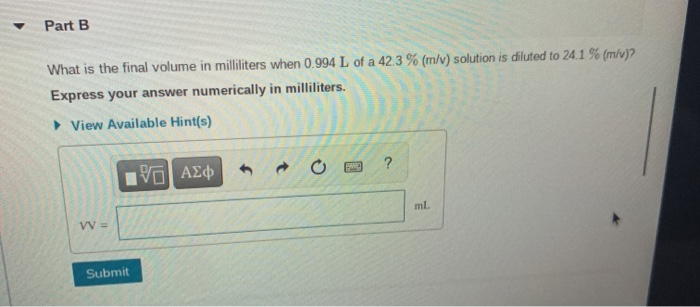Part B What is the final volume in milliliters when 0.994 L of a 42.3 % (m/v) solution is diluted to 24.1 % (m/v)? Express your answer numerically in milliliters. View Available Hint(s) ? Pa| ΑΣφ ml W = Submit 7 of 28 A Review Constants Periodic Table Part C A 894 ml NaCl solution is diluted to a volume of 1.02 L and a concentration of 6.00 M. What was the initial concentration? Express the molar concentration numerically. View...

• ### M Review | Constants | Periodic Table - Part A When a solution contains a weak...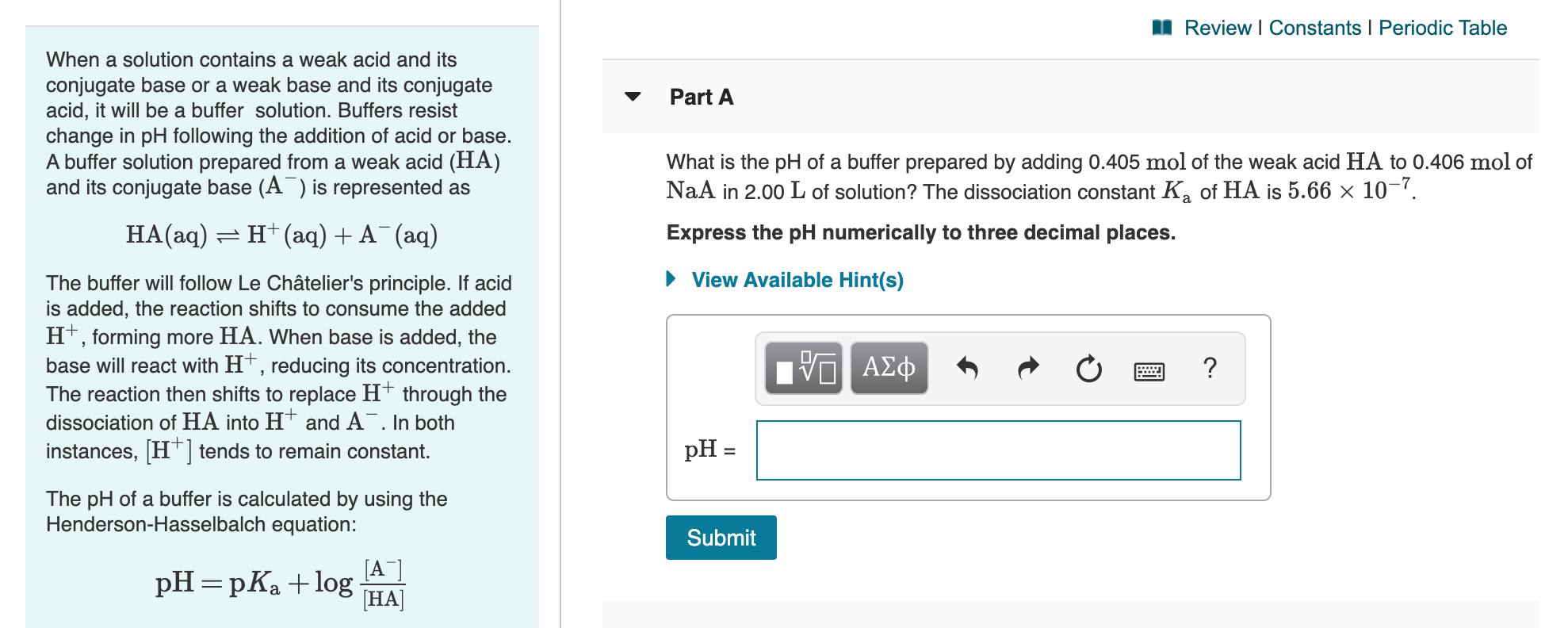M Review | Constants | Periodic Table - Part A When a solution contains a weak acid and its conjugate base or a weak base and its conjugate acid, it will be a buffer solution. Buffers resist change in pH following the addition of acid or base. A buffer solution prepared from a weak acid (HA) and its conjugate base (A) is represented as What is the pH of a buffer prepared by adding 0.405 mol of the weak acid...

• ### C 7 of 16 A Review Constants Periodic Table Part A is stable bon-14, a As...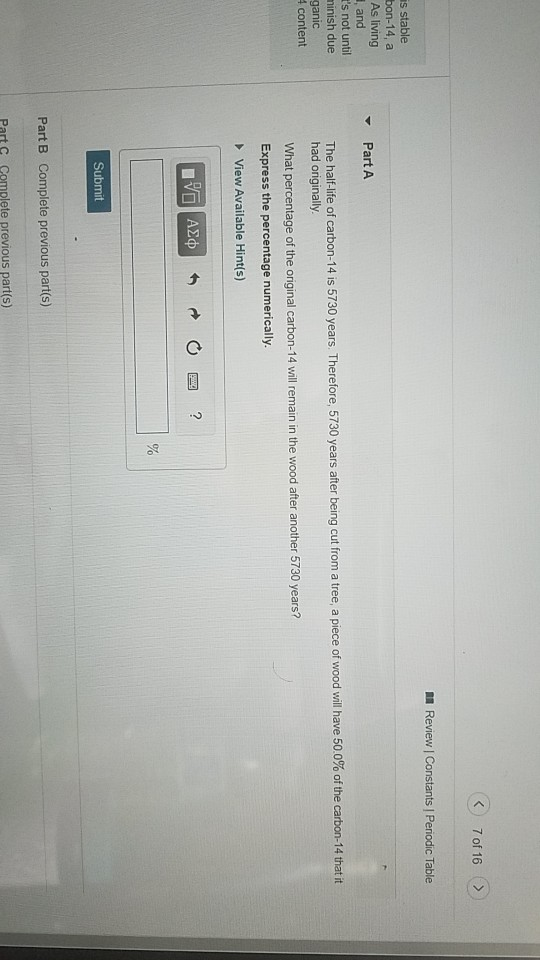C 7 of 16 A Review Constants Periodic Table Part A is stable bon-14, a As living and t's not until minish due ganic 4 content The half-life of carbon-14 is 5730 years. Therefore, 5730 years after being cut from a tree, a piece of wood will have 50.0% of the carbon-14 that it had originally What percentage of the original carbon-14 will remain in the wood after another 5730 years? Express the percentage numerically. ► View Available Hint(s) IVO...

• ### Review Constants 1 Periodic Table The concentration of OH in a sample of seawater is 1.2x10-6...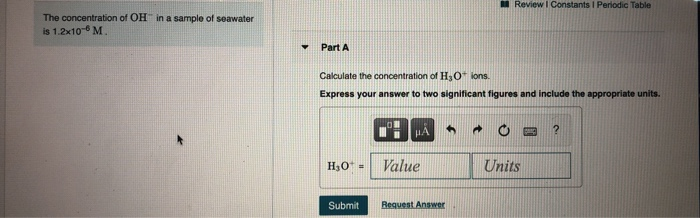Review Constants 1 Periodic Table The concentration of OH in a sample of seawater is 1.2x10-6 M Y Part A Calculate the concentration of H3O+ ions. Express your answer to two significant figures and include the appropriate units. HA ? H0+ - Value Units Submit Request Answer Review Constants ! Periodic Table Water Ionizes by the equation Part A H2O(1) H+ (aq) + OH(aq) The extent of the reaction is small in pure water and dilute aqueous solutions. This reaction...

• ### < on 10 of 28 A Review Constants Periodic Table Part A What is the boiling...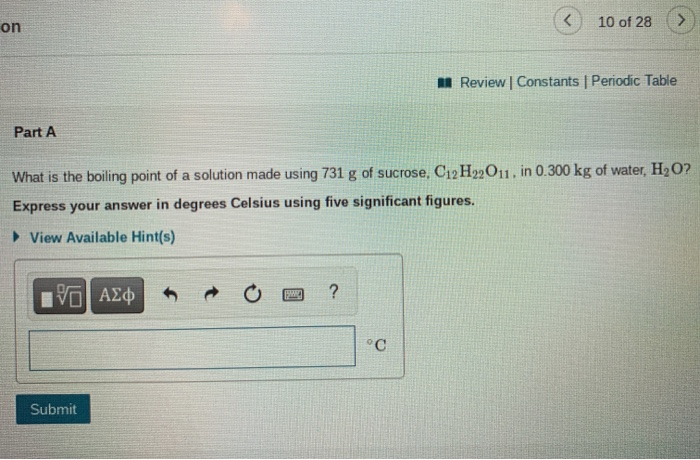< on 10 of 28 A Review Constants Periodic Table Part A What is the boiling point of a solution made using 731 g of sucrose, C12H22011. in 0.300 kg of water, H2O? Express your answer in degrees Celsius using five significant figures. View Available Hint(s) VO AO ? °C Submit Part B What is the freezing point of a solution that contains 26.4 g of urea, CO(NH2)2. in 225 mL water, H2O? Assume a density of water of 1.00...

• ### Review I Constants 1 Periodic Table Part B What is the final volume V2 in milliliters...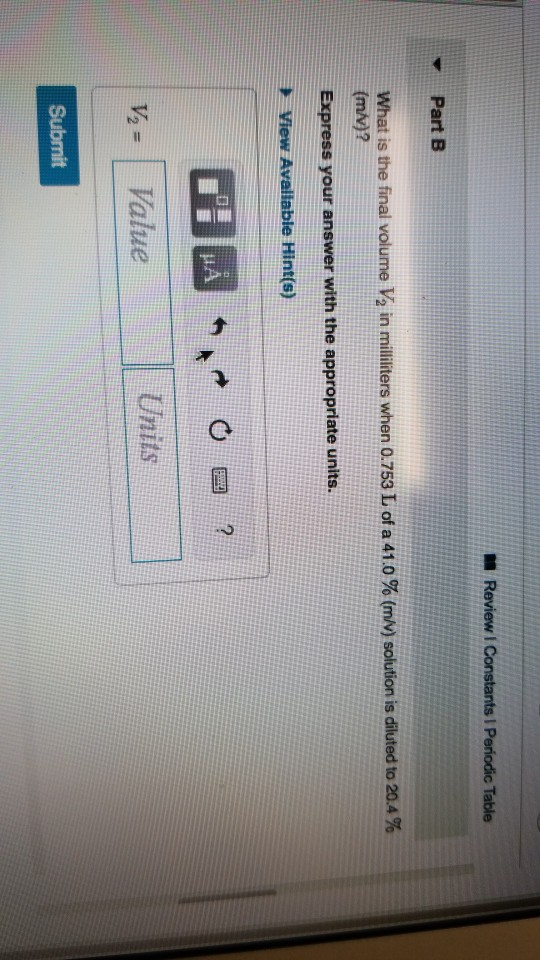Review I Constants 1 Periodic Table Part B What is the final volume V2 in milliliters when 0.753 L of a 41.0 % (m/v) solution is diluted to 20.4 % (m/v)? Express your answer with the appropriate units. View Available Hint(s) DA *MAN OR? Value Units V = Submit Review | Constants 1 Periodic Table Part C A 841 ml NaCl solution is diluted to a volume of 1.46 L and a concentration of 8.00 M. What was the initial...